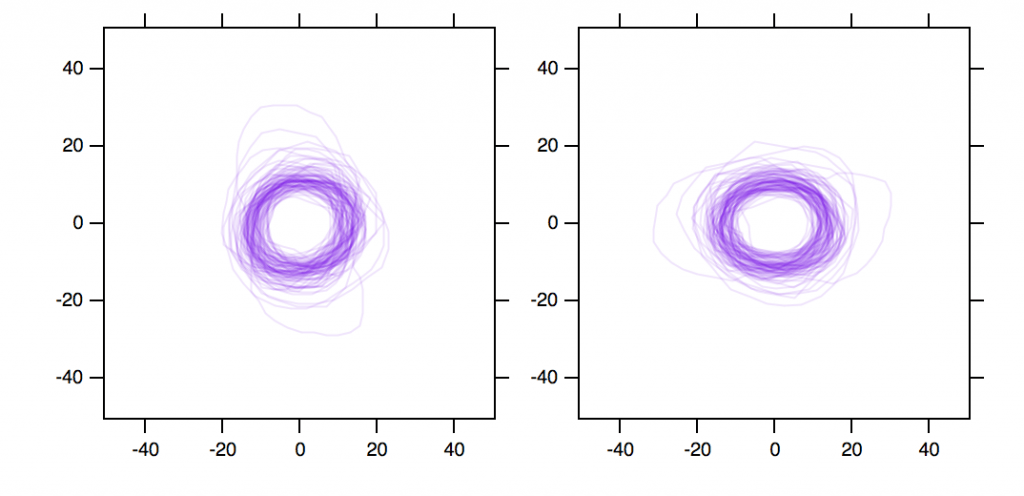# Esoteric Circle

Many projects in the lab involve quantifying circular objects. Microtubules, vesicles and so on are approximately circular in cross section. This quick post is about how to find the diameter of these objects using a computer.

So how do you measure the diameter of an object that is approximately circular? Well, if it was circular you would measure the distance from one edge to the other, crossing the centre of the object. It doesn’t matter along which axis you do this. However, since these objects are only approximately circular, it matters along which axis you measure. There are a couple of approaches that can be used to solve this problem.

Principal component analysis

The object is a collection of points* and we can find the eigenvectors and eigenvalues of these points using principal component analysis. This was discussed previously here. The 1st eigenvector points along the direction of greatest variance and the 2nd eigenvector is normal to the first. The order of eigenvectors is determined by their eigenvalues. We use these to rotate the coordinate set and offset to the origin.Now the major axis of the object is aligned to the x-axis at y=0 and the minor axis is aligned with the y-axis at x=0 (compare the plot on the right with the one on the left, where the profiles are in their original orientation – offset to zero). We can then find the absolute values of the axis crossing points and when added together these represent the major axis and minor axis of the object. In Igor, this is done using a oneliner to retrieve a rotated set of coords as the wave M_R.

PCA/ALL/SEVC/SRMT/SCMT xCoord,yCoord

To find the crossing points, I use Igor’s interpolation-based level crossing functions. For example, storing the aggregated diameter in a variable called len.

FindLevel/Q/EDGE=1/P m1c0, 0
len = abs(m1c1(V_LevelX))
FindLevel/Q/EDGE=2/P m1c0, 0
len += abs(m1c1(V_LevelX))

This is just to find one axis (where m1c0 and m1c1 are the 1st and 2nd columns of a 2-column wave m1) and so you can see it is a bit cumbersome.

Anyway, I was quite happy with this solution. It is unbiased and also tells us how approximately circular the object is (because the major and minor axes tell us the aspect ratio or ellipticity of the object). I used it in Figure 2 of this paper to show the sizes of the coated vesicles. However, in another project we wanted to state what the diameter of a vesicle was. Not two numbers, just one. How do we do that? We could take the average of the major and minor axes, but maybe there’s an easier way.

Polar coordinates

The distance from the centre to every point on the edge of the object can be found easily by converting the xy coordinates to polar coordinates. To do this, we first find the centre of the object. This is the centroid $$(\bar{x},\bar{y})$$ represented by

$$\bar{x} = \frac{1}{n}\sum_{i=1}^{n} x_{i}$$ and $$\bar{y} = \frac{1}{n}\sum_{i=1}^{n} y_{i}$$

for n points and subtract this centroid from all points to arrange the object around the origin. Now, since the xy coords are represented in polar system by

$$x_{i} = r_{i}\cos(\phi)$$ and $$y_{i} = r_{i}\sin(\phi)$$

we can find r, the radial distance, using

$$r_{i} = \sqrt{x_{i}^{2} + y_{i}^{2}}$$

With those values we can then find the average radial distance and report that.

There’s something slightly circular (pardon the pun) about this method because all we are doing is minimising the distance to a central point initially and then measuring the average distance to this minimised point in the latter step. It is much faster than the PCA approach and would be insensitive to changes in point density around the object. The two methods would probably diverge for noisy images. Again in Igor this is simple:

Make/O/N=(dimsize(m1,0)-1)/FREE rW

rW[] = sqrt(m1[p]^2 + m1[p]^2)

len = 2 * mean(rW)
Here again, m1 is the 2-column wave of coords and the diameter of the object is stored in len.

How does this compare with the method above? The answer is kind of obvious, but it is equidistant between the major and minor axes. Major axis is shown in red and minor axis shown in blue compared with the mean radial distance method (plotted on the y-axis). In places there is nearly a 10 nm difference which is considerable for objects which are between 20 and 35 nm in diameter. How close is it to the average of the major and minor axis? Those points are in black and they are very close but not exactly on y=x.So for simple, approximately circular objects with low noise, the ridiculously simple polar method gives us a single estimate of the diameter of the object and this is much faster than the more complex methods above. For more complicated shapes and noisy images, my feeling is that the PCA approach would be more robust. The two methods actually tell us two subtly different things about the shapes.

Why don’t you just measure them by hand?

In case there is anyone out there wondering why a computer is used for this rather than a human wielding the line tool in ImageJ… there are two good reasons.

1. There are just too many! Each image has tens of profiles and we have hundreds of images from several experiments.
2. How would you measure the profile manually? This approach shows two unbiased methods that don’t rely on a human to draw any line across the object.

* = I am assuming that the point set is already created.

The post title is taken from “Esoteric Circle” by Jan Garbarek from the LP of the same name released in 1969. The title fits well since this post is definitely esoteric. But maybe someone out there is interested!